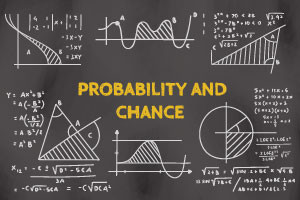Probability and Chance in Mathematics | Online Maths Course | Alison# Probability and Chance in Mathematics - Revised

## This free online math course from Alison introduces you to the mathematics of probability and chance.

Probability and Statistics
Free Course
This free online mathematics course will be a massive help to any student studying mathematical probability and chance. It covers topics from calculating the probability of simple events to Bayes Theorem and Binomial distribution. You will also look into Bernoulli Trials and the binomial Formula, study the use of Excel to calculate binomial probabilities, learn to find the mean of a binomial distribution, and a whole lot more!

1.5-3 Hours

Yes

Yes

Yes
• ### Publisher

XSIQ## Description

This Probability and Chance in Mathematics course introduces you to the mathematics of probability and chance. The course starts off by teaching you about making arrangements and selection in mathematics. It then covers how to calculate expected values.

Next you will learn about calculating the probability of both simple and compound events, along with probability in independent events. You will then be introduced to tree diagrams and how to calculate probability with them. You will also learn about calculating probability in reverse using Bayes Theorem.

The second module starts off by teaching you about what distributions are and how to compare them. You will then learn about what binomial distribution is and how to work out a maths problem using binomial distribution. Finally, you will be taught how to use Microsoft Excel to calculate monomial probabilities and the mean of a binomial distribution.

Start Course Now

## Learning Outcomes

Having completed this course students will be able to:

• Discuss and calculate the mathematical ways making arrangement and selections
• Recognise the concept of Expected value
• Discuss and define what probability is
• Calculate the probability of simple and compound events
• Discuss how to calculate probability in reverse
• Recognize Bayes Theorem
• Identify how to calculate the probability distribution
• Define what a Binomial Distribution is and how to calculate it
• Identify how to calculate the normal distribution for continuous variables

## Certification

All Alison courses are free to enrol, study and complete. To successfully complete this Certificate course and become an Alison Graduate, you need to achieve 80% or higher in each course assessment. Once you have completed this Certificate course, you have the option to acquire an official Certificate, which is a great way to share your achievement with the world. Your Alison Certificate is:

Ideal for sharing with potential employers - include it in your CV, professional social media profiles and job applications
An indication of your commitment to continuously learn, upskill and achieve high results
An incentive for you to continue empowering yourself through lifelong learning

Alison offers 3 types of Certificates for completed Certificate courses:

Digital Certificate - a downloadable Certificate in PDF format, immediately available to you when you complete your purchase
Certificate - a physical version of your officially branded and security-marked Certificate, posted to you with FREE shipping
Framed Certificate - a physical version of your officially branded and security-marked Certificate in a stylish frame, posted to you with FREE shipping